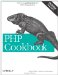# Recipe 2.2. Comparing Floating-Point Numbers

#### 2.2.1. Problem

You want to check whether two floating-point numbers are equal.

#### 2.2.2. Solution

Use a small delta value, and check if the numbers have a difference smaller than that delta:

`<?php \$delta = 0.00001; \$a = 1.00000001; \$b = 1.00000000; if (abs(\$a - \$b) < \$delta) { /* \$a and \$b are equal */ } ?>`

#### 2.2.3. Discussion

Floating-point numbers are represented in binary form with only a finite number of bits for the mantissa and the exponent. You get overflows when you exceed those bits. As a result, sometimes PHP (just like some other languages) doesn't believe that two equal numbers are actually equal because they may differ toward the very end.

To avoid this problem, instead of checking if \$a == \$b, make sure the first number is within a very small amount (\$delta) of the second one. The size of your delta should be the smallest amount of difference you care about between two numbers. Then use abs( ) to get the absolute value of the difference.

Recipe 2.3 for information on rounding floating-point numbers; documentation on floating-point numbers in PHP at http://www.php.net/language.types.float.PHP Cookbook: Solutions and Examples for PHP Programmers
ISBN: 0596101015
EAN: 2147483647
Year: 2006
Pages: 445

Similar book on Amazon